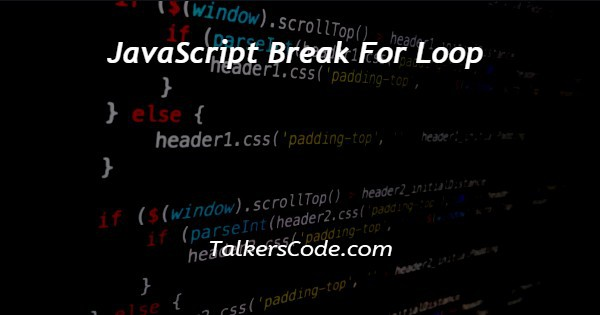# JavaScript Break For Loop

Last Updated : Aug 29, 2023In this article we will show you the solution of JavaScript break for loop, JavaScript's powerful 'break' statement is a control flow element that gives programmers the ability to modify loop behaviour. It offers a way to leave a loop early under specific circumstances.

In this article, we will look towards how we use the “break” statement for improving performance of our code.

In this tutorial, we will examine the "break" statement and learn how to use it in various loop structures, including the "for," "while," and "do-while" loops.

We'll go over several real-world situations to show how employing the "break" statement can result in more understandable and concise code.

In this tutorial, we will take a practical approach and provide clear and short code examples to demonstrate how to use the 'break' statement.

We'll start by outlining the fundamental structure and operation of the "break" statement within various kinds of loops.

Then, using useful code blocks, we'll demonstrate how it may be used in actual situations.

## Step By Step Guide On JavaScript Break For Loop :-

```const n = [2, 9, 14, 21, 5, 28];
let result = -1;
for (let i = 0; i < n.length; i++) {
if (n[i] % 7 === 0) {
result = n[i];
break;
}
}
console.log("The first multiple of 7 is:", result);```
1. Initializing of constant array n: [2, 9, 14, 21, 5, 28] in first step.
2. Result is a declared variable with a -1 initial value. The array n's first multiple of 7 will be stored in this variable.
3. The elements of the array n are iterated through using a for loop in the code.
4. The initialization statement let i = 0 sets the loop counter variable i to 0 at the beginning of the loop.
5. As long as the expression i n.length, where n.length denotes the number of elements in the array n, is true, the loop will keep running.
6. The code determines whether the current element n[i] is a multiple of 7 by checking the if condition n[i]% 7 === 0 after each iteration. When n[i] is divided by 7, the remainder is determined using the % operator. If there is no leftover, then n[i] must be a multiple of 7.
7. The value of n[i] is assigned to the result variable, and the loop is ended with the break statement if the test n[i]% 7 === 0 is successful. This makes sure that the result only contains the first multiple of 7 that was discovered in the array.
8. The loop continues to the following iteration with a result of -1 if the condition n[i]% 7 === 0 is not met (i.e., n[i] is not a multiple of 7).
9. Using the console.log("The first multiple of 7 is:", result) command, the value of result got printed when loop got finished which represents the first multiple of 7 in the given array n.

## Conclusion :-

In this article, we had looked towards how we use the JavaScript 'break' command and how it get used in various looping.

For increasing efficiency of our code, we use the ‘break’ statement which enables us to quit loops early depends on our certain conditions.

It is an excellent tool in a developer's toolbox and can aid in writing code that is shorter and more efficient.

To prevent potential problems in your programmes, utilise it sparingly and make sure the loops you break out of are properly constructed.

I hope this article on JavaScript break for loop helps you and the steps and method mentioned above are easy to follow and implement.